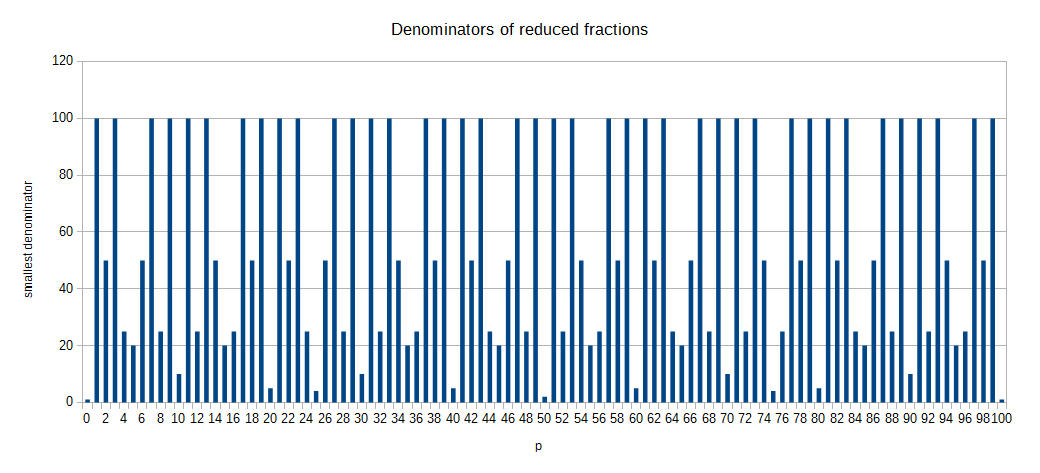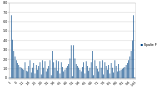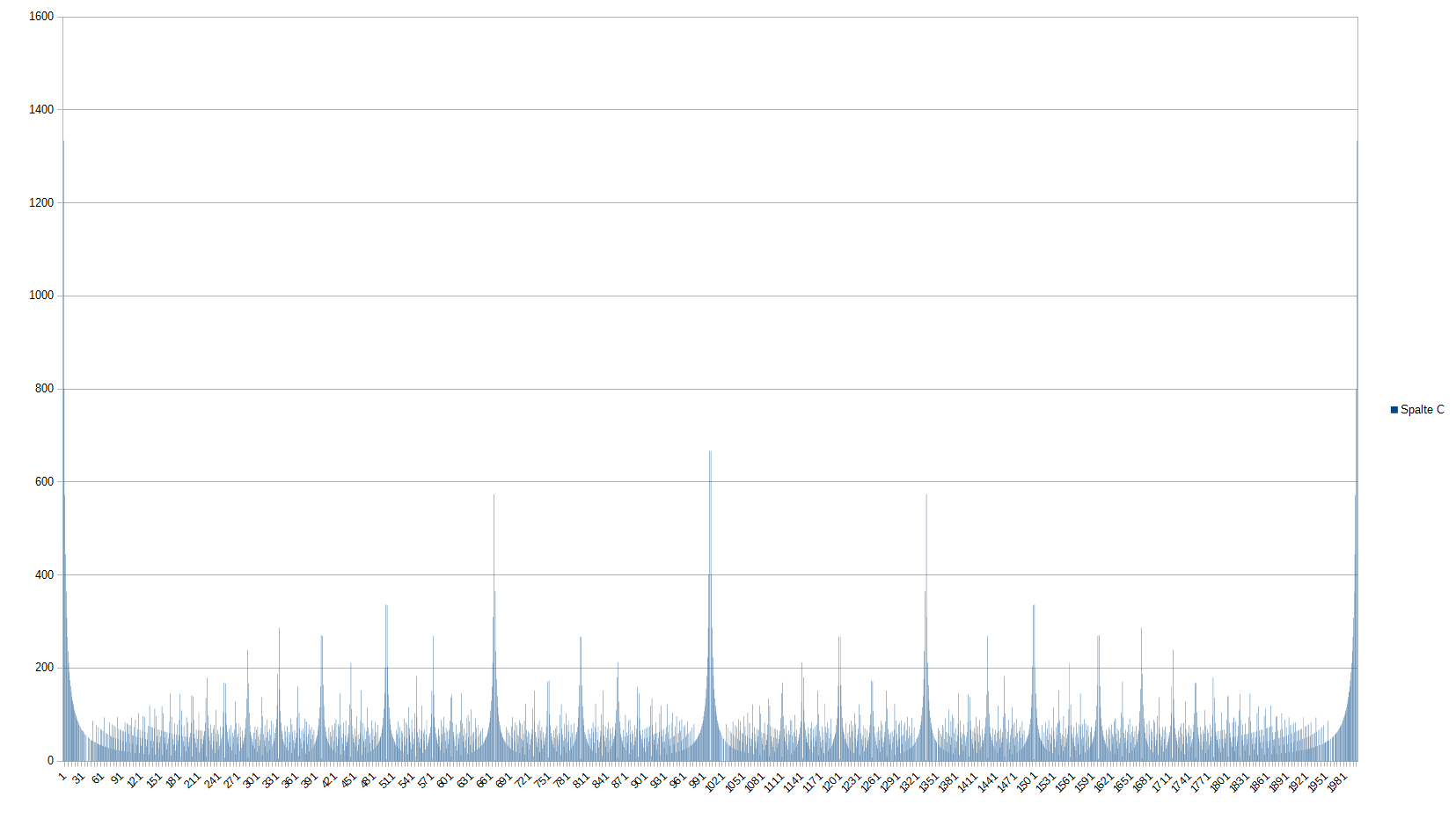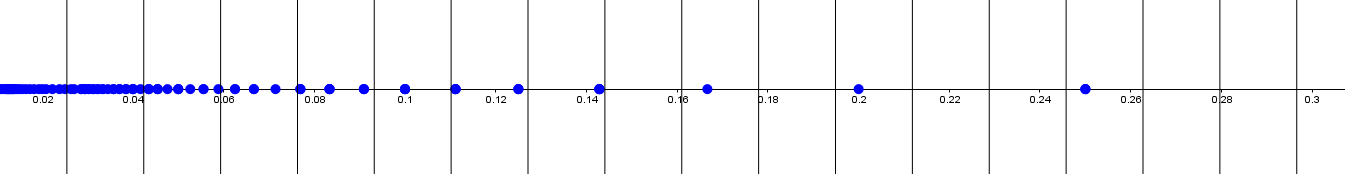# Percentages and decimals as rounded fractions

Related problem

Of course, every possible integer percentage $0 \leq p \leq 1, 100p \in \mathbb{N}$ can be expressed as a fraction $\frac a {100}, a=100p$. Many of these fractions (actually 61, because $61 = 101 - \phi(100) = 101 - 40$ with Euler's totient function $\phi(n)$) can be reduced to have a smaller denominator.However, if we allow rounding, we can express most percentages with even smaller denominators, e.g. $17\% \approx \frac 1 6$.

Rounding

First of all, we have to define how to round. There are many ways to do this, but we have to conditions that characterize the method we'll use.

• Every number $p$ that isn't already an integer (in this case we wouldn't have to round) has two neighbouring integers. Unless the fractional part of $p$, $p-\lfloor p \rfloor$ is $0.5$ we can just round to the nearest integer.
• When working with fractions, there is a nice symmetry $\frac a b + \frac {b-a} b = 1$. This seems trivial, but we definitely want to keep it. So $\frac 1 8 + \frac 7 8$ should equal $1$. But when rounded, we get $0.13 + 0.88 = 1.01$. So, to preserve symmetry, we will always round away from $0.5$. $0.125 ≈ 0.12$, but $0.875 ≈ 0.88$.

These two conditions characterize our rounding method, and we can also write a formula that satisfies both:

$p \approx \frac 1 {100} ( \text{sgn} (100p-50) \lfloor | 100p-50 | + \frac1 2 \rfloor +50 )$

The sign-function $\text{sgn} (x)$ gives $1$ if $x$ is nonnegative and $-1$ otherwise.

If we now want to round with some other precision $a \in \mathbb{N}$, we can use the formula

$p \stackrel{s}\approx \frac 1 s \left( \text{sgn} (sp-\frac s 2) \lfloor | sp-\frac s 2 | + \frac1 2 \rfloor + \frac s 2 \right)$

To give another notation, let's define

$r_s(p) = \frac 1 s \left( \text{sgn} (sp-\frac s 2) \lfloor | sp-\frac s 2 | + \frac1 2 \rfloor + \frac s 2 \right)$

and in particular

$r(p) = r_{100}(p)$

The smallest possible denominator

With these rules in mind, we can now look at the topic of this note – expressing decimals (like percentages) as rounded fractions.

As common, we will only consider reduced fractions; and in this part mainly their denominators.

Let us define a function $q_s(p)$ to give the smallest denominator $q$ for which there exists a numerator $a$ so that $\frac a q$ equals $p$ when rounded with precision $s$.

$q_s(p) = \min \{q \in \mathbb{N} : (\exists a \in \mathbb{N})[r_s(\frac a q) = p] \}$

Now we can explore some properties of $q_s(p)$.

If we graph $q_s(p)$ with some fixed precision, say $s=100$, we see some interesting patterns.

Every $p$ that can be written with a small denominator $q$ has neighbours that need very large denominators. For example, $p=0.5 = \frac 1 2 \Rightarrow q_{100}(0.5)=2$, but $q_{100}(0.49) = 35$. This is because for every other $q < 35$, either the fraction could be reduced to exactly $\frac 1 2$ or $\frac 1 q$ just didn't make small enough steps to hit $0.49$.

We can make a table of $q_s(p)$ when $s$ goes to infinity and $p$ is very small, so it's influenced by $0$.

$\displaystyle \lim_{s \to \infty}q_s(1) = \left\lceil \frac 2 3 s \right\rceil$

$\displaystyle \lim_{s \to \infty}q_s(2) = \left\lceil \frac 2 5 s \right\rceil$

And in general,

$\displaystyle \lim_{s \to \infty}q_s(p) = \left\lceil \frac 2 {2p+1} s \right\rceil$

Influence

To formalize this influence of simple fractions, let's define a funtion $i_s(p)$ to give the number of values from $p$ upward for which $q$ is decreasing.

$i_s(p) = max\{ p_k : sp_k \in \mathbb{N} \land q_s\left(p+\frac 1 s\right)>q_s\left(p+\frac 2 s\right)>\cdots>q_s\left(p+\frac k s\right) \} - p$

We can look at another diagram of $q_s(p)$ with bigger $s$, in this case $s=2000$) to see how $i_s(p)$ behaves.There are all those spikes marking the neighbours of simple fractions, and it somehow looks a little bit like a fractal.

The influence of $0$ is easiest to calculate. It can be done with a computer program, but there is no closed formula since it really depends on the value of $s$.

pseudocode:

 1 2 3 4 5 6 if (x=0) (f(1/s, s)) else if (1/floor(1/x)>x+1/(2*s) and 1/ceiling(1/x)<=x-1/(2*s)) x else f(x+1/s, s) 

What we can do, is give an approximation for $i(0, s)$ when $s$ gets very large. Since the influence counts the number of $p$-values whose denominators are decreasing, it will stop at the first fraction with a higher denominator, say $p_1$. But the one before it, $p_0$, had a smaller denominator and was smaller. So it necessarily also had a smaller numerator, actually $1$ because otherwise it could have been replaced by some other fraction with numerator $1$ and smaller denominator. From all this we get that the first fraction $p_1$ to interrup the sequence has to have a numerator greater than $1$. This means that there is no fraction with numerator $1$ that would get rounded to $p_1$, so it's too far away from any unit fraction.

We can imagine regions in which all numbers get rounded to the same number as a grid with each cell spanning $\frac 1 s$ units.This is the grid for $s=59$. Every point marks one unit fraction and will get rounded to the center of the interval it is in. We notice that between $0.14$ and $0.16$ there is an empty interval. This corresponds to the fact that no unit fraction will get rounded to approximately $0.15$$\frac 1 6$ is too big and $\frac 1 7$ too small. So actually, we just have to find the first interval without any unit fractions and then we can calculate $i(0, s)$.

Finding the empty interval

To find an empty interval, we have to compare the size of the intervals (which is constant and equals $\frac 1 s$) with the size of the gaps between unit fractions.

The difference between some unit fraction $\frac 1 n$ and the next one, $\frac 1 {n+1}$ is given by

$\frac 1 n - \frac 1 {n+1} = \frac 1 {n(n+1)} = \frac 1 {n^2+n}$

If this gap is greater than $\frac 1 s$, this doesn't imply that the interval is empty. $\frac 1 n$ could still be in the interval, $\frac 1 {n+1}$ in the previous and $\frac 1 {n-1}$ in the next.

What is sufficient, is that the gap is greater than $\frac 2 s$. A unit fraction could still be in this interval, but either the previous or the next have to leave an empty interval. To find an $n$ that satisfies this, we set up the inequality

\begin{aligned} \frac 1 {n^2+n} &\geq \frac 2 s \\ n^2+n &\leq \frac s 2 \\ n^2+n-\frac 1 2 s &\leq 0 \\ n &\leq \frac {-1 \pm \sqrt{1+2s}}{2} \\ n &\leq \frac {\sqrt{2s+1}-1}{2} \end{aligned}

At first this looks like an upper bound, but actually it is our lower bound for $n$ or upper bound for $\frac 1 n$. This is because the inequality gives us a sufficient condition, so there could be smaller poasible values for $n$. We have to remember that and use it later. Now, we also have to find a upper bound for $n$.

If the gap between two unit fractions is less than $\frac 1 s$, the spacing of our intervals, then there has to be a unit fraction in every interval. So we can set up a second inequality

\begin{aligned} \frac 1 {n^2+n} &< \frac 1 s \\ n^2+n &> s \\ n^2+n-s &> 0 \\ n &> \frac {-1 \pm \sqrt{1+4s}}{2} \\ n &> \frac {\sqrt{4s+1}-1}{2} \\ \end{aligned}

This is also actually an upper bound, because it is a sufficient condition that there is nos empty interval for all $n$ values. Ther could be a gap for $n$ greater than that.

If we now combine the two, rembering to swap the inequality signs, we find the following conditions for $n$

$\frac {\sqrt{4s+1}-1}2 \geq n > \frac{\sqrt{2s+1}-1}2$

Somewhere in this region there will be some $n$ that works. When $s$ gets very large, we can say

$\frac {\sqrt{4s+1}-1}2 \sim \sqrt{s}$

and

$\frac {\sqrt{2s+1}-1}2 \sim \frac 1 {\sqrt{2}} \sqrt{s}$

But, for the influence we care about $\frac 1 n$ and we have to multiply by $s$ to get the number of influenced $p$s, not the value. So we see, when $s$ is large,

$\sqrt{s} < i(0, s) < \sqrt{2}\sqrt{s}$

Variables and functions I used

Variables

• $a, b, c, d \in \mathbb{N}$: temporary variables
• $k$: some index
• $0 \leq p \leq 1, p \in \mathbb{R}$: the value we want to express
• $s \in \mathbb{N}$: the precision of our rounding
• $q \in \mathbb{N}$: the smallest possible denominator
• $i, j$: the influence

Functions

• $\lfloor \cdot \rfloor$: The floor function
• $\text{sgn} (\cdot)$: The sign function
• $|\cdot|$: the absolute valueNote by Henry U
1 year, 3 months ago

This discussion board is a place to discuss our Daily Challenges and the math and science related to those challenges. Explanations are more than just a solution — they should explain the steps and thinking strategies that you used to obtain the solution. Comments should further the discussion of math and science.

When posting on Brilliant:

• Use the emojis to react to an explanation, whether you're congratulating a job well done , or just really confused .
• Ask specific questions about the challenge or the steps in somebody's explanation. Well-posed questions can add a lot to the discussion, but posting "I don't understand!" doesn't help anyone.
• Try to contribute something new to the discussion, whether it is an extension, generalization or other idea related to the challenge.

MarkdownAppears as
*italics* or _italics_ italics
**bold** or __bold__ bold
- bulleted- list
• bulleted
• list
1. numbered2. list
1. numbered
2. list
Note: you must add a full line of space before and after lists for them to show up correctly
paragraph 1paragraph 2

paragraph 1

paragraph 2

[example link](https://brilliant.org)example link
> This is a quote
This is a quote
    # I indented these lines
# 4 spaces, and now they show
# up as a code block.

print "hello world"
# I indented these lines
# 4 spaces, and now they show
# up as a code block.

print "hello world"
MathAppears as
Remember to wrap math in $$ ... $$ or $ ... $ to ensure proper formatting.
2 \times 3 $2 \times 3$
2^{34} $2^{34}$
a_{i-1} $a_{i-1}$
\frac{2}{3} $\frac{2}{3}$
\sqrt{2} $\sqrt{2}$
\sum_{i=1}^3 $\sum_{i=1}^3$
\sin \theta $\sin \theta$
\boxed{123} $\boxed{123}$

Sort by:

Epidermal Growth Factor Receptor (EGFR) is a transmembrane glycoprotein with molecular weights ranging from 170 to 185 KDa, expressed in some normal epithelial, mesenchymal, and neurogenic tissue. And EFGR is one of the four members of the ErbB family tyrosine kinase receptors. It consists of a C-terminus intracellular region that possesses the kinase activity, and an N-terminus extracellular ligand-binding site, a hydrophobic transmembrane domain.

https://www.creative-biogene.com/crispr-cas9/solution/egfr-gene-editing.html

- 3 months ago

Does anyone know how to define a function $i(p, s)$ in real code, maybe in Python?

 if (p=0) then (i(1/s, s)) else if (1/floor(1/p)>p+1/(2*s) and 1/ceiling(1/p)<=x-1/(2*s)) then p else i(p+1/s, s)

- 1 year, 3 months ago

Henry, you switched the bracket signs for the links and AWESOME NOTE!!!!

- 1 year, 3 months ago

Thank you!! It's my first note of this kind and I'm glad you like it.

- 1 year, 3 months ago

Actually, if you progress at this rate, YOU ARE A LEGEND!

- 1 year, 3 months ago

Thank you SOO much!!

- 1 year, 3 months ago

You put in so much effort and you dare say that this is your first note! Imagine you are a seasoned note writer! LEGEND!

- 1 year, 3 months ago